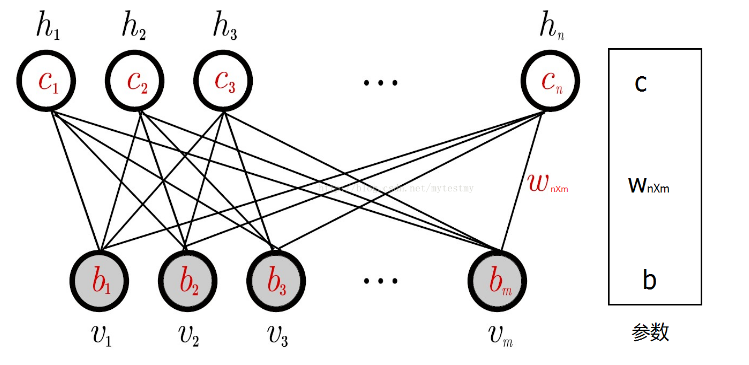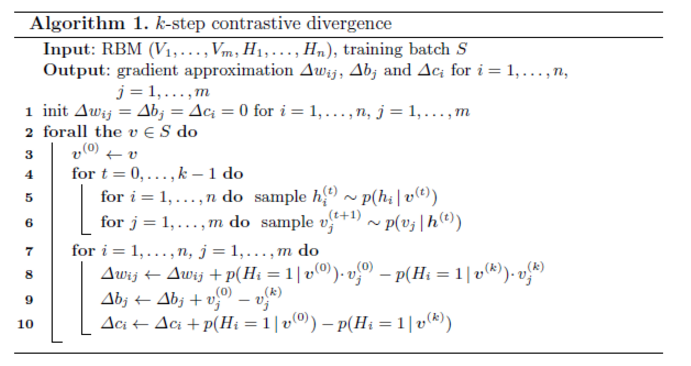# RBM(Restricted Bolzmann Machines)原理

## 能量模型

p(x)=eE(x)Z

Z$Z$为配分函数：
Z=xeE(x)

L(θ,D)=1Nx(i)Dlogp(x(i))

l(θ,D)=L(θ,D)

## 能量模型的作用

1. RBM属于一种无监督学习的方法，无监督学习的目的是最大可能地拟合训练数据。
2. 对于一组输入数据来说，如果我们不知道它属于什么分布，我们是非常难学习的。如，如果我们知道数据符合高斯分布，我们就可以确定参数，利用极大似然即可轻松地学习，但如果不知道什么分布，就无从入手。但是，统计力学的结论表明，任何概率分布都可以转换成能量模型。，这为拟合任何数据带来了可行性。
3. 在马尔科夫随机场(MRF)中，能量模型充当了两个角色：一、全局解的度量(目标函数)；二、能量最小时的解为目标解。这为能量模型提供了目标函数和目标解。

## RBM结构### 编码过程

1. 根据模型可以计算出隐藏层节点i取值为1的条件概率p(hi=1|v)$p(h_i=1|v)$
2. 根据p(hi=1|v)$p(h_i=1|v)$这个概率来随机选择hi$h_i$的取值为1还是0。
3. 对每个隐层节点执行上述操作。

### 解码过程

1. 根据模型可以计算出可视层节点i取值为1的条件概率p(vi=1|h)$p(v_i=1|h)$
2. 根据p(vi=1|h)$p(v_i=1|h)$这个概率来随机选择vi$v_i$的取值为1还是0。
3. 对每个隐层节点执行上述操作。

### 概率的计算

E(v,h)=i=1nj=1mwijhivjj=1mbjvji=1ncihi

p(v,h)=eE(v,h)v,heE(v,h)

P(v)=heE(v,h)v,heE(v,h)

P(h)=veE(v,h)v,heE(v,h)

P(v|h)=eE(v,h)veE(v,h)

P(h|v)=eE(v,h)heE(v,h)

### 极大似然

RBM求解的目标就是——让RBM网络表示的Gibbs分布最大可能的拟合训练数据（分布）。

L(θ,D)=1Nx(i)Dlogp(x(i))

### 求解极大似然

L(θ)θ=vlnp(v)θ

lnp(x)=lnheE(v,h)v,heE(v,h)=ln(heE(v,h))ln(v,heE(v,h))

lnp(x)θ=heE(v,h)(E(v,h)θ)heE(v,h)v,heE(v,h)(E(v,h)θ)v,heE(v,h)=h(p(h|v)(E(v,h)θ))v,h(p(v,h)(E(v,h)θ))=Ep(h|v)[E(v,h)θ]Ep(h,v)[E(v,h)θ]

lnp(x)wij=h(p(h|v)(E(v,h)wij))v,h(p(v,h)(E(v,h)wij))=hp(h|v)hivjv,hp(v,h)hivj=hp(h|v)hivjvp(v)hp(h|v)hivj=p(hi=1|v)vjvp(v)p(hi=1|v)vj

lnp(x)bj=h(p(h|v)(E(v,h)bj))v,h(p(v,h)(E(v,h)bj))=hp(h|v)vjv,hp(v,h)vj=hp(h|v)vjvp(v)hp(h|v)vj=vjvp(v)vj

lnp(x)ci=h(p(h|v)(E(v,h)ci))v,h(p(v,h)(E(v,h)ci))=hp(h|v)hiv,hp(v,h)hi=hp(h|v)hivp(v)hp(h|v)hi=p(hi=1|v)vp(v)p(hi=1|v)

p(vj=1|h)=vkjp(vj=1,vkj,h)vp(v,h)=σ(i=1nwijhi+bj)

p(hi=1|v)hkip(hi=1,hki,v)hp(v,h)=σ(j=1mwijvj+ci)

lnp(x)wij=p(hi=1|v)vjvp(v)p(hi=1|v)vj=p(hi=1|v)vj1lk=1lp(hi=1|vyk)vyk

lnp(x)bj=vjvp(v)vj=vj1lk=1lvyk

lnp(x)ci=p(hi=1|v)vp(v)p(hi=1|v)=p(hi=1|v)1lk=1lp(hi=1|vyk)

### 抽样方法

h0p(h|v0)$h_0 \sim p(h|v_0)$ v1p(v|h0)$v_1 \sim p(v|h_0)$
h1p(h|v1)$h_1 \sim p(h|v_1)$ v2p(v|h1)$v_2 \sim p(v|h_1)$
$……，$ vk+1p(v|hk)$v_{k+1} \sim p(v|h_k)$